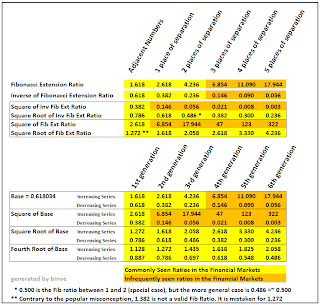I analyze macroeconomic issues from a fundamental perspective, and I analyze market behavior from a technical perspective. Original macroeconomic analysis can be found here and both macro analysis and commentary can be found on my Caps blog. If you like or appreciate my analysis, please add yourself to my Following List

## Thursday, October 27, 2011

### Randomly Useful: Fibonacci Ratio Table (Updated)

This is just a mostly trivial update to the original post: Randomly Useful: Fibonacci Ratio Table. Added another calculation variant to derive a few more ratios and to show the coolness of the Fibonacci relationships via the fact that they can be arrived at multiples ways.

And no, just adding 1 or 2 to a ratio is *not* a valid technique to derive ratios (and yes, I am speaking to all the knuckleheads who still use 1.382 as a Fibonacci ratio)

------------------------------------

Nothing Earth-shattering, but here is a nice little table of Fibonacci Ratios. I actually generated this table a long time ago (6 months?, 9 months? edit 10/27/11: Okay, lets just say a few years ago :) ). Anyways, I would sometimes come across lists of Fib ratios. But as a guy who wants to understand where things come from in the first place, that was very unsatisfying.

The are many ways to come up with Fibonacci ratios besides looking at adjacent Fib numbers. There is the exact equation, which gives you the main one (0.618 and 1.618). But the most clever way is as a system of linear equations (and since I love linear algebra, this is very cool). I was planning on writing a really detailed post of why I love phi and going on a geek trip using linear equations, like I did regarding e as with this post: Why e is the coolest number. But I decided to restrain myself this time :)

Anyways, here is the table. Have fun! :)